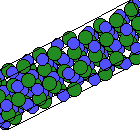Crystal Lattice Structures: Creation Date:  1 Jul 2001 Last Modified: 21 Oct 2004

# The Moissanite-9R CSi Crystal StructureYou can now

• This is a hypothetical alternate stacking (ABCBCACAB) for tetrahedral structures. Compare this to Zincblende (ABCABC), Wurtzite (ABABAB), and 6H (ABCACB). The 9R refers to the fact that there are 9 CSi dimers in a hexagonal unit cell (which contains 3 rhombohedral unit cells). Zincblende is denoted 3C, and Wurtzite is 2H.
• This structure is related to the αSm (C19) structure in the same way that Zincblende (B3) is related to the fcc (A1) lattice.
• The spacings in structure file assume that the local environment is similar to Zincblende CSi. Thus the internal parameters have the values given in the table below, and c/a = (9/2) (8/3)½.

• Prototype: CSi
• Pearson Symbol: hR6
• Space Group: R3m (Cartesian and lattice coordinate listings available)
• Number: 160
• Primitive Vectors:
• a and c are the lattice constants of the equivalent hexagonal unit cell.
 A1 = + ½ a X + ½ 3-1/2 a Y + 1/3 c Z A2 = - ½ a X + ½ 3-1/2 a Y + 1/3 c Z A3 = - 3-1/2 a Y + 1/3 c Z
• Basis Vectors:
• All atoms are on (1a) Wyckoff positions. The 'ideal' structure parameters, those that get us closest to the Zincblende structure, are:  x1 = 0 x2 = - 2/9 x3 = + 2/9 x4 = 3/36 x5 = 11/36 x6 = -5/36
 B1 = x1 (A1 + A2 + A3) = x1 c Z (C-I) (1a) B2 = x2 (A1 + A2 + A3) = x2 c Z (C-II) (1a) B3 = x3 (A1 + A2 + A3) = x3 c Z (C-III) (1a) B4 = x4 (A1 + A2 + A3) = x4 c Z (Si-I) (1a) B5 = x5 (A1 + A2 + A3) = x5 c Z (Si-II) (1a) B6 = x6 (A1 + A2 + A3) = x6 c Z (Si-III) (1a)

Go back to the carbon structures page.

Go back to Crystal Lattice Structure page.

 Structures indexed by: This is a mirror of an old page created at theNaval Research LaboratoryCenter for Computational Materials ScienceThe maintained successor is hosted at http://www.aflowlib.org/CrystalDatabase/ and published as M. Mehl et al., Comput. Mater. Sci. 136 (Supp.), S1-S828 (2017).